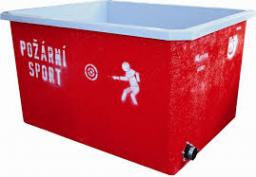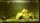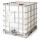# Fire tank

How deep is the fire tank with the dimensions of the bottom 7m and 12m, when filled with 420 m3 of water?

h =  5 m

### Step-by-step explanation:Did you find an error or inaccuracy? Feel free to write us. Thank you!Tips to related online calculators
Tip: Our volume units converter will help you with the conversion of volume units.

## Related math problems and questions:

• TankTo cuboid tank whose bottom has dimensions of 9 m and 15 m were flow 1080 hectoliters of water. This was filled 40% of the tank volume. Calculate the depth of the tank.
• Water tank300hl of water was filled into the tank 12 m long and 6 m wide. How high does it reach?
• Fire tank1428 hl of water is filled in a block-shaped fire tank with the edges of the base 12 m and 7 m. Calculate the content of water-wetted areas.
• Water tankA 288 hectoliter of water was poured into the tank with dimensions 12 m and 6 m bottom and 2 m depth. What part of the volume of the tank water occupied? Calculate the surface of tank wetted with water.
• The rainwaterThe rainwater container has the shape of a block whose bottom has dimensions of 4.5 m and 3.5 m. It is partly filled with water. What is the level if there is 189 hl of water in it?
• Tank full2 hl of water were poured into a block-shaped tank with dimensions of 450 mm and 600 mm and a height of 900 mm. How many liters of water need to be added to keep the tank full?
• TankThe tank bottom has dimensions of 1.5 m and 3 2/6 m. The tank is 459.1 hl of water. How high is the water surface?
• Water tankWater tank shape of cuboid has dimensions of the bottom 7.5 meters and 3 meters. How high will reach the water in the tank will flow 10 liters of water per second and the inflow will be open 5/6 hour? (Calculate to one decimal place and the results give i
• Water tankWhat is the height of the cuboid-shaped tank with the bottom dimensions of 80 cm and 50 cm if the 480 liters of water reach 10 cm below the top?
• Water poolWhat water level is in the pool shaped cuboid with bottom dimensions of 25 m and 10 meters, when is 3750hl water in the pool.
• TankHow many minutes does it take to fill the tank to 25 cubic meters of water filled 150hl per hour?
• TrayWhat height reach water level in the tray shaped a cuboid, if it is 420 liters of water and bottom dimensions are 120 cm and 70 cm.
• Swimming poolThe pool shape of a cuboid is 299 m3 full of water. Determine the dimensions of its bottom if the water depth is 282 cm and one bottom dimension is 4.7 m greater than the second.
• Third dimensionCalculate the third dimension of the cuboid: a) V = 224 m3, a = 7 m, b = 4 m b) V = 216 dm3, a = 9 dm, c = 4 dm
• The potThe pot is in 1/3 filled with water. Bottom of the pot has an area of ​​329 cm2. How many centimeters rises water level in the pot after add 1.2 liters of water?
• The poolThe cube-shaped pool has 140 cubic meters of water. Determine the dimensions of the bottom if the depth of the water is 200 cm and one dimension of the bottom is 3 m greater than the other. What are the dimensions of the pool bottom?
• WaterInto a full cylindrical tank high 3 m with a base radius of 2.5 m, we insert cuboid with dimensions 1.7 m, 1.3 m, 1.9 m. How many liters of water will overflow out?